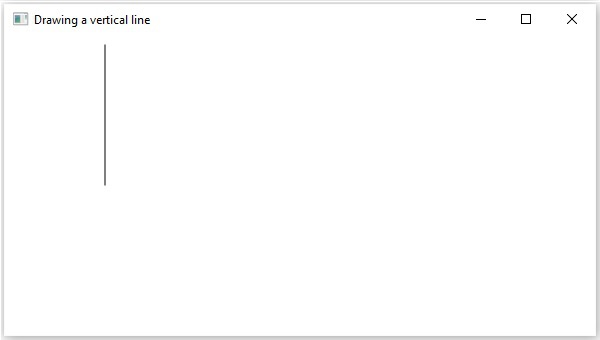# 2D Shapes PathElement Vertical Line

The path element Vertical Line is used to draw a vertical line to a point in the specified coordinates from the current position.

It is represented by a class named VLineTo. This class belongs to the package javafx.scene.shape.

This class has a property of the double datatype namely −

• Y − The y coordinate of the point to which a vertical is to be drawn from the current position.

To draw the path element vertical line, you need to pass a value to this property. This can be done either by passing it to the constructor of this class at the time of instantiation as follows −

```LineTO line = new LineTo(x)
```

Or, by using its respective setter methods as follows −

```setY(value);
```

## Steps to draw PathElement Vertical Line

To draw a vertical line to a specified point from the current position in JavaFX, follow the steps given below.

### Step 1: Creating a Class

Create a Java class and inherit the Application class of the package javafx.application and implement the start() method of this class as follows.

```public class ClassName extends Application {
@Override
public void start(Stage primaryStage) throws Exception {
}
}
```

### Step 2: Create the path class object

Create the path class object as follows −

```//Creating a Path object
Path path = new Path()
```

### Step 3: Create a Path

Create the MoveTo path element and set XY coordinates to the starting point of the line to the coordinates (100, 150). This can be done by using the methods setX() and setY() of the class MoveTo as shown below.

```//Moving to the starting point
MoveTo moveTo = new MoveTo();
moveTo.setX(100.0f);
moveTo.setY(150.0f)
```

### Step 4: Creating an object of the class VLineTo

Create the path element vertical line by instantiating the class named VLineTo, which belongs to the package javafx.scene.shape as follows.

```//Creating an object of the class VLineTo
VLineTo vLineTo = new VLineTo();
```

### Step 5:Setting Properties to the Element Vertical Line

Specify the coordinates of the point to which a vertical line is to be drawn from the current position. This can be done by setting the properties x and y using their respective setter methods as shown in the following code block.

```//Setting the Properties of the vertical line element
lineTo.setX(500.0f);
lineTo.setY(150.0f);
```

### Step 6: Adding Elements to the Observable List of the Path Class

Add the path elements MoveTo and VlineTo created in the previous steps to the observable list of the Path class as follows −

```//Adding the path elements to Observable list of the Path class
```

### Step 7: Creating a Group Object

Create a group object by instantiating the class named Group, which belongs to the package javafx.scene.

Pass the Line (node) object created in the previous step as a parameter to the constructor of the Group class. This can be done in order to add it to the group as shown below −

```Group root = new Group(line);
```

### Step 8: Creating a Scene Object

Create a Scene by instantiating the class named Scene which belongs to the package javafx.scene. To this class, pass the Group object (root) created in the previous step.

In addition to the root object, you can also pass two double parameters representing height and width of the screen along with the object of the Group class as follows −

```Scene scene = new Scene(group ,600, 300);
```

### Step 9: Setting the Title of the Stage

You can set the title to the stage using the setTitle() method of the Stage class. The primaryStage is a Stage object which is passed to the start method of the scene class as a parameter.

Using the primaryStage object, set the title of the scene as Sample Application as follows.

```primaryStage.setTitle("Sample Application");
```

### Step 10: Adding Scene to the Stage

You can add a Scene object to the stage using the method setScene() of the class named Stage. Add the Scene object prepared in the previous steps using this method as shown below −

```primaryStage.setScene(scene);
```

### Step 11: Displaying the Contents of the Stage

Display the contents of the scene using the method named show() of the Stage class as follows.

```primaryStage.show();
```

### Step 12: Launching the Application

Launch the JavaFX application by calling the static method launch() of the Application class from the main method as follows.

```public static void main(String args[]){
launch(args);
}
```

### Example

Following is a program which draws a vertical line from the current point to a specified position using the class Path of JavaFX. Save this code in a file with the name − VLineToExample.java.

```import javafx.application.Application;
import javafx.scene.Group;
import javafx.scene.Scene;
import javafx.stage.Stage;
import javafx.scene.shape.VLineTo;
import javafx.scene.shape.MoveTo;
import javafx.scene.shape.Path;

public class VLineToExample extends Application {
@Override
public void start(Stage stage) {
//Creating an object of the Path class
Path path = new Path();

//Moving to the starting point
MoveTo moveTo = new MoveTo();
moveTo.setX(100.0);
moveTo.setY(150.0);

//Instantiating the VLineTo class
VLineTo vLineTo = new VLineTo();

//Setting the properties of the path element vertical line
vLineTo.setY(10.0);

//Adding the path elements to Observable list of the Path class

//Creating a Group object
Group root = new Group(path);

//Creating a scene object
Scene scene = new Scene(root, 600, 300);

//Setting title to the Stage
stage.setTitle("Drawing a vertical line");

stage.setScene(scene);

//Displaying the contents of the stage
stage.show();
}
public static void main(String args[]){
launch(args);
}
}
```

Compile and execute the saved java file from the command prompt using the following commands.

```javac VLineToExample.java
java VLineToExample
```

On executing, the above program generates a JavaFX window displaying a vertical line, which is drawn from the current position to the specified point, as shown below.javafx_2d_shapes.htm